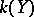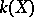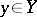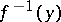# Zariski theorem

Jump to: navigation, search

on connectivity, Zariski connectedness theorem

Letbe a proper surjective morphism of irreducible varieties, let the field of rational functionsbe separably algebraically closed inand letbe a normal point; thenis connected (moreover, geometrically connected) (see ). The theorem provides a basis for the classical principle of degeneration: If the generic cycle of an algebraic system of cycles is a variety (i.e. is geometrically irreducible), then any specialization of that cycle is connected.

A special case of the Zariski connectedness theorem is the so-called fundamental theorem of Zariski, or Zariski's birational correspondence theorem: A birational morphism of algebraic varietiesis an open imbedding into a neighbourhood of a normal pointifis a finite set (see ). In particular, a birational morphism of normal varieties which is bijective at points is an isomorphism. Another formulation of this theorem: Letbe a quasi-finite separable morphism of schemes, and letbe a quasi-compact quasi-separable scheme; then there exists a decomposition, whereis a finite morphism andan open imbedding .